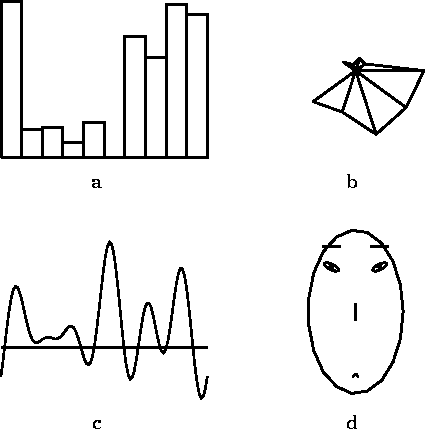Next: Clustering methods Up: METHODS FOR EXPLORATORY DATA Previous: METHODS FOR EXPLORATORY DATA

## Visualization of high-dimensional data items

Several graphical means have been proposed for visualizing high-dimensional data items directly, by letting each dimension govern some aspect of the visualization and then integrating the results into one figure (cf., e.g., du Toit et al., 1986; Jain and Dubes, 1988). These methods can be used to visualize any kinds of high-dimensional data vectors, either the data items themselves or vectors formed of some descriptors of the data set like the five-number summaries [Tukey, 1977].

Perhaps the simplest method to visualize a data set is to plot a ``profile'' of each item, i.e., a two-dimensional graph in which the dimensions are enumerated on the x-axis and the corresponding values on y (Fig. 1 a). An alternative is a scatterplot where two original dimensions of the data are chosen to be portrayed as the location of an icon, and the rest of the dimensions are depicted as properties of the icon. For example the lengths of rays emanating from the center of the icon may visualize the values of the rest of the components (Fig. 1 b). Also the familiar pie diagrams can be used.Figure 1: A ten-dimensional data item visualized using four different methods. a A profile of the component values, b a ``star'' in which the length of each ray emanating from the center illustrates one component, c Andrews' curve, and d a facial caricature.

Andrews' curves [Andrews, 1972], one curve for each data item, are obtained by using the components of the data vectors as coefficients of orthogonal sinusoids, which are then added together pointwise (Fig. 1 c).

Chernoff's faces [Chernoff, 1973] are among the most famous visual displays. Each dimension of the data determines the size, location, or shape of some component of a facial caricature (Fig. 1 d). For example, one component is associated with the width of the mouth, another with the separation of the eyes, etc.

The major drawback that applies to all these methods in the data mining setting is that they do not, used as such, reduce the amount of data. If the data set is large, the display consisting of all the data items portrayed separately will be incomprehensible. The methods could, however, be useful for illustrating some kinds of summaries of the data set like the cluster centroids that are introduced below, or the reference vectors of a self-organizing map.Next: Clustering methods Up: METHODS FOR EXPLORATORY DATA Previous: METHODS FOR EXPLORATORY DATA